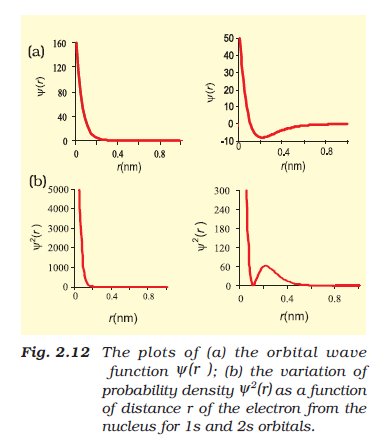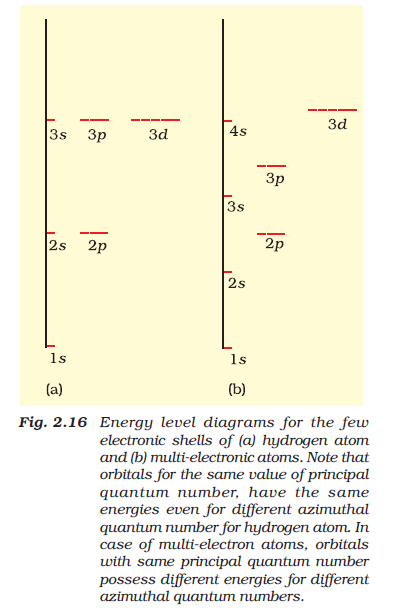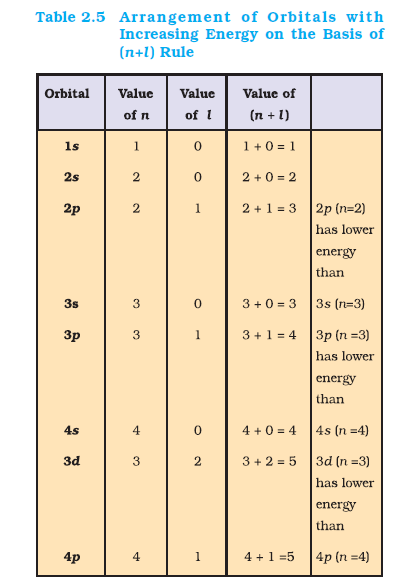Chemistry Shape of Atomic Orbitals
Click for Only Video

### Topics to be covered :

● Shapes of Atomic Orbitals.
● Boundary Surface Diagram.
● Angular Nodes .
● Degenerate Orbitals .
● Energy of Orbitals.
● Nodal surface or Node .
● Reason.

### Shapes of Atomic Orbitals=> psi has no physical meaning. It is simply a mathematical function of the coordinates of the electron. Fig 2.12(a)

=> According to Max Born, the square of wave function(i.e,  psi^2) at a point gives the probability density of the electron at that point. Fig 2.12(b).

=> For 1s probability density is maximum at the nucleus and it decreases sharply as we move away from it. But for 2s orbital the probability density first decreases sharply and again starts increasing. After reaching a maxima it decreases again and approaches zero as the value of r further increases.

### Nodal surface or Node :

The region where the probability density function reaches to zero.

For ns-orbital, there are (n - 1) nodes,

Representation of probability density curve : -

The probability density variation can be imagined in terms of charge could diagrams [fig 2.13 (a)]

### Boundary Surface Diagram :This gives fairly good representation of the shapes of the orbitals.

In this, a boundary surface or contour surface is drawn in space for an orbital on which the value |psi|^2  is constant.

Definition : For a given orbital, only that boundary surface diagram of constant probability density is taken which encloses a region or volume in which the probability of finding the electron is 90%. fig [2.13 (b)].

Note : - We do not draw boundary surface diagram of 100% probability because  psi^2 has always some value at any finite distance from the nuclues, so it is not possible to draw a boundary surface diagram of a rigid size of 100% probability.

For s-orbital, boundary surface diagram is spherical in shape. i.e the probability of finding the electron in all direction is equal.

Size of s-orbital prop 'n'

For p-orbital (Fig 2.14), the boundary surface diagram is not spherical. But it has two lobes which are on either side of the plane passing through the nucleus. At this plane, probability density function is zero.

The shape, size and energy of the three orbitals are identical. The lobes lie along the x, y and z axis and they are given the designations 2p_x, 2p_y and 2p_z.

For p-orbital, the energy of p-orbitals prop n

For d-orbitals, l = 2. And there are five d-orbitals, designated as d_(xy), d_(yz), d_(zx), d_(x^2-y^2) and d_(z^2). See fig 2.15.

All the five d-orbitals have same energy.

### Angular Nodes :

The probability density functions at the plane passing through the nuecleus is zero. This is called angular node.

No. of angular nodes = l

Total no. of nodes = n-1

No. of radial nodes  = (n-l-1)
Q 2612045839The nodes present in 3p - orbitals are

(A)two spherical

(B)two planar

(C)one planar

(D)one planar one sphericalSolution:

The nodes present in 3p-orbitals are one spherical and one planar.
Correct Answer is => (D) one planar one spherical

### Energies of Orbitals :Energy of an electron in a H-atom is determined by n.

The order of energy follows as

1s < 2s = 2p < 3s = 3p = 3d < 4s = 4p = 4d = 4f < ................

See fig.2.16

Note : The 2s and 2p - oribitals have same energy even though their shape is different.

### Degenerate Orbitals :

Orbitals having same energy are called degenerate orbitals.

The 1s-orbital corresponds to the most stable condition and is called the ground state.

The electron is this orbital is most strongly held by the nucleus.

Electron of H-atom present in 2s, 2p or higher orbitals is said to be in excited state.

The energy of an electron in a multi-electron atom depends on both n and l.

### Reason :The energy of an electron in a multi-electron atom depends on both n and l the mutual repulsion among the electrons.

The reason of stability of multi-electron system is

text(total attractive interaction > total repulsive interactions)

Repulsive interaction is among the electrons of outer shell and inner shell.

Attractive interaction is between the electrons and nucleus.

As the positive charge (Ze) on the nucleus increases, the attractive interaction increases.

Due to the presence of inner electrons, outer electrons will not experiene the full positive charge of the nucleus (Ze).

This is known as the shielding of the outer shell electrons from (the nucleus) by the inner shell electrons, and the net positive charge which outer electrons experience is known as the effective nuclear charge (Z_text(eff) e).

Both attractive and repulsive interaction depends upon the shell and shape of the orbital in which the electron is present.

s-orbitals have the most effective shielding power.

The order of shielding power is :

s > p > d > f

Within a shell, s-orbital electron spends more time closer to the nucleus compared to other orbitals electrons. The order of this is given by

s > p > d > f

The more time a orbital electron spends near the nucleus, the more tightly it is bound to the nucleus.

The energy of the s-orbital will be lower i.e. more stable than p-orbital.

Due to different shielding effect of different orbitals, it leads to the difference in energy of orbitals in same shell.

The lower the value of (n+l) for an orbital, the lower is its energy. See Table 2.5.

If two orbitals have same value of (n+l), then the orbital having lower n value will have lower energy.

Energies of the orbitals in the same subshell decreases with increase in the atomic number..

e.g. E_(2s) (H) > E_(2s) (Li) > E_(2s) (Na) > E_(2s) (K)
Q 1946078873Among the following pairs of orbitals which
orbital will, experience the larger effective
nuclear charge?

(i) 2s and 3s
(ii) 4d and 4f
(iii) 3d and 3p
Class 11 Exercise 2 Q.No. 64Solution:

(i) 2s is closer to the nucleus than 3s. Hence
2s will experience larger effective nuclear
charge.
(ii) 4d
(iii) 3p (for same reason)